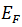# Occupation Probability - Engineering Physics

Occupation Probability

According to classical theory all the electrons in a metal can have the same energy, so that, at 0K, they all condense into the lowest available energy. But when quantum statistics is applied we find that these electrons occupy states between the energy values O and.

Fermi has shown that the probability of occupancy of a particular quantum state is given as

is the Fermi energy at temperature T. The function (1) is plotted in Fig. for various values of T.

Let us consider the behavior of Fermi factor at different temperature -

, the Fermi distribution becomes identical with that of Boltzman distribution. The meaning of this is that all the states beloware not filled and hence all the states above the relation (1.74) does not remain valid and the entire distribution becomes Maxwell-Boltzmann. This is shown for temperaturein Fig.